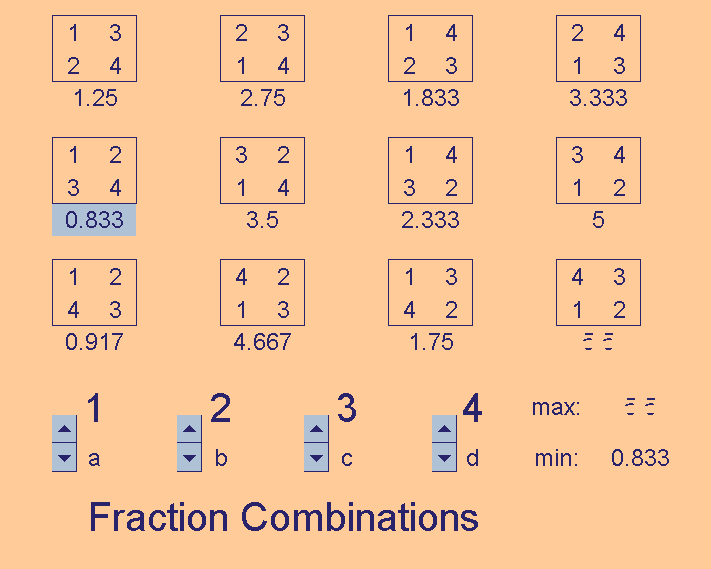# Excel Investigation: Fraction Combinations

##### Age 11 to 16Challenge Level

Take four numbers say 2, 5, 7, 15.
Use the four numbers to make two fractions, for example: 2/5 and 7/15.

There are 12 ways you could have done that.

For the numbers a, b, c, and d, the value a could go with the b, c, or d.
That's 3 choices. The un-chosen letters then make the other pair without any further choice.
For each of the two pairs there are 2 possibilities, the numbers could be either way up: a/b or b/a.

So 3 . 2 . 2 = 12 arrangements.

Which of these arrangements gives the greatest (or least) sum (or difference)? That's four questions.

Can you generalise, producing a process or strategy that will always identify the combination required for the fractions to be as far apart as possible?The Excel file Fraction Combinations shows the twelve arrangements.
You can experiment with different values for a, b, c, and d.
The combination producing the greatest and least result colour automatically so as to be conspicuous.
When you open the Fraction Combinations file you will see a text box explaining how to change the Excel formula to investigate the difference rather than the sum of the fractions.

Remember for most of the Excel resources it's best to save the file rather than open it directly from the hyperlink.

To do this: right-click the link, choose Save Target As, then select the directory (folder) in which you wish the file to be saved.